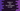# Python program to find out the third largest number in a list### Python 3 program to find out the third-largest number in a list :

In this python tutorial, we will learn how to find out the third largest number in a list. For example the third largest number among 1,5,4,2,7,9 is 5. Our program will iterate through the list only for one time i.e. the time complexity of this program is O(n).

### Python program :

``````#1
num = [2,3,7,4,5,6,10,11,120]

#2
largest_num = num
second_largest_num = num
third_largest_num = num

#3
for i in num :
#4
if i > largest_num :
third_largest_num = second_largest_num
second_largest_num = largest_num
largest_num = i
#5
elif i > second_largest_num :
third_largest_num = second_largest_num
second_largest_num = i
#6
elif i > third_largest_num :
third_largest_num = i

#7
print("Third largest number of the list is {}".format(third_largest_num))``````

### Explanation :

The commented numbers in the above program denote the step numbers below:

1. First, create one number list holding few random numbers. We will find the third largest among these numbers.
2. Create three variables to hold the largest number,second largest number, and third largest number of the list. Assign them all the value of the first number of the given list.
3. Run one for loop to read each number of the list one by one.
4. First, check if the current number is greater than the largest number. If yes, assign the value of the second-largest number to the third-largest number, the value of the largest number to the second-largest number, and the current value of the number to the largest number. Basically, we are updating the value for these three variables.
5. If the first condition failed, check if it is larger than the second-largest number or not. If yes, assign the value of the second-largest number to the third-largest number and assign the current reading value to the second largest number.
6. Similarly, check if the current number is only greater than third-largest number. If yes, assign its value to the third-largest number variable.
7. Finally, print out the result, i.e. the value of the third largest number variable.

### Output :

``Third largest number of the list is 10``